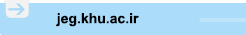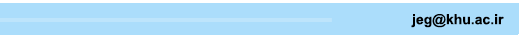[Home ] [Archive]   [ فارسی ]Main About Current Issue Archive Search Submit ContactVolume 14, Issue 2 (8-2020)2020, 14(2): 283-308 Back to browse issues page
Estimation of Soil-Pile Stiffness under the Bridge Piers Considering Soil-Structure Interaction using Artificial Neural Network Model
1- , hoseinishima1365@gmail.com
2- Khajeh Nasir Toosi University, Tehran, Iran
Abstract:   (1845 Views)
Introduction
The discussion of modeling the interaction of soil-pile groups due to a large number of parameters involved in is one of the complex topics and it has been one of the interests to researchers in recent years and has been dealt with in various ways. In recent years, the artificial neural network method has been used in many issues related to geotechnical engineering, including issues related to piles.. In this study, firstly it was tried to explain the importance of soil - structure interaction in calculating the dynamic response of bridges. Then, the effect of different effective parameters in calculating the interaction stiffness of the pile - soil group using artificial neural network was studied.  For this purpose, firstly, Sadr Bridge ( The intersection of Modarress and Kaveh Boulevard because the presence of tallest piers ) in the transverse direction, considering and without considering of the effect of soil - structure interaction was analyzed. The analysis was carried out in which the substructure soil was replaced with a set of springs and dashpots along the piles. Considering the fact that many factors are involved in determining the equivalent stiffness of springs, in the second stage, the effect of different factors on the stiffness of spring equations using artificial neural network was investigated. Finally, the artificial neural network method was used as a suitable method in order to estimate the equivalent stiffness values, the equivalent stiffness of the pile - soil group was introduced for different input values. equivalent stiffness of the substructure soil using the artificial neural network ,has not been used by researchers yet, so estimation of the optimal length and diameter of piles used in constructions and estimating the seismic performance of the bridge system after its implementation could be effective .
Material and methods
In this paper, spring-dashpot method is proposed to the non-uniform analysis of single-pier bridges which led to a 5-degree freedom model in the case of Sadr Bridge. This study also endeavors to investigate the SSI effect in dynamic analysis of bridges. This method is based on the traditional spring-dashpot method but in this method, non-linear stiffness is used along the piles, instead of linear stiffness and upgraded shape functions and coefficients are applied to make more precise mass, stiffness and damping matrices. Then the seismic responses of Sadr Bridge are compared in different conditions including or excluding the SSI effects. Considering the fact that in the present study to calculate the stiffness of the soil-pile group at depth, due to the effect of soil - structure interaction, the recommended method by API is used, the study of neural network analysis was used and the effect of different parameters used to determine the complexity of the soil-pile group system has been evaluated. The multi-layer feeder network, which has the most application in engineering issues, has an input layer, an output layer and one or more layers of hidden content, has been used for this purpose.  The best model of the neural network with a topology of 1-20-6 was provided using the hyperbolic sigmoid activation function, and the Levenberg Marquardt model and the training cycle 84, which had the least error mean square and the best regression coefficient. The effect of internal friction angle, soil density, pile diameter and the resistance per unit length has been evaluated with this method.
Results and discussion
 ارائه شده است صورت می پذیرد In this study, the importance of considering the effect of soil - structure interaction on the dynamic response of the Sadr Bridge was studied. Dynamic stiffness of the soil around the pile group was calculated based on the equivalent linear method and using the p-y springs. So, the effect of substructure soil was considered in dynamic analysis of the system . The artificial neural network was used to predict the stiffness of the soil - pile group, based on various input parameters and the stiffness sensitivity analysis of the calculated output values was conducted. In hard soils, the stiffness of the pile - soil group increases with increasing the diameter of the pile in the range of 1 to 1.5 m in diameter. However, in the range of 0.5 to 1 m in diameter, the diameter of the pile does not have much effect on the stiffness of the system and also stiffness decreases in the range of 1.5 to 2 m in diameter by increasing the pile diameter. Soil specific weight and angle of internal friction can change the system stiffness but the effect of the soil specific density is much greater on the stiffness of the soil-pile group system. Generally, the specific density in the range of 1000 to 2300 (kg/m3) will increase the stiffness of the system. In general, the ultimate strength of the soil among 100 to 550 (kN/m) affects the system stiffness. This effect within the ultimate strength between 100 and 220 (kN/m) causes increasing in the interaction stiffness value of the system and in the range of 220 to 550 (kN/m) causes reducing the stiffness of the system . The ultimate strength values ​​in a unit of length outside of the above range have little effect on the system interference stiffness. Despite the fact that the problem of calculating the soil - pile interaction stiffness is a direct solution, the use of the proposed neural network model can help in predicting optimal values ​​of diameter and length of the pile to achieve maximum soil- pile stiffness and especially for long bridges it will has a significant impact on reducing cost and seismic design of the bridge.
Conclusion
The results of this study are as follows:
The results showed that considering the interaction effect, although it increases the relative displacement of the deck, reduces the maximum base shear and moment. This suggests that considering the maximum base shear and moment in the interaction conditions may not lead to a seismic design for certainty, although closer to reality.
Artificial neural network is an efficient way and new method to predict the stiffness of the soil-pile group system based on different input values that have not been used yet. So that with the physical and mechanical properties of the soil as well as the geometric properties of the piles, it is possible to predict the interaction stiffness values with the proper precision.
According to the results and diagrams obtained from the neural network model, which are mainly sinusoidal, the optimal values ​​of the interaction stiffness can be obtained by obtaining the pile diameter, specific gravity, the internal soil friction soil to achieve optimal interaction strength. It is also possible for each site to estimate the depth of the piles in order to achieve optimal hardness.
./files/site1/files/142/4Extended_Abstracts.pdf
Keywords: soil interaction - pile group, artificial neural network, dynamic response, single-pillar bridge
Type of Study: s | Subject: Geotecnic
Received: 2018/09/7 | Accepted: 2019/01/5 | Published: 2020/08/31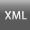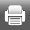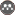Rights and permissionsThis work is licensed under a Creative Commons Attribution-NonCommercial 4.0 International License.
 Rights and permissionsThis work is licensed under a Creative Commons Attribution-NonCommercial 4.0 International License.